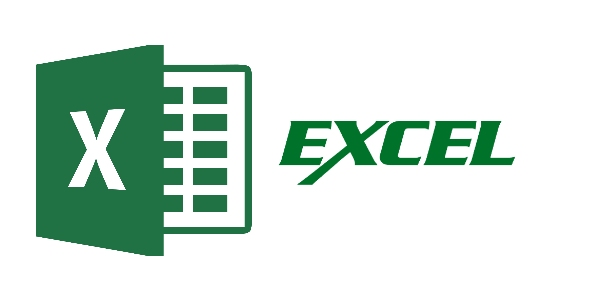# Excel Advanced Questions By Akshay Magre

20 Questions | Attempts: 337SettingsThis Set of Questions are for Target Audience already aware of Basic Excel Concepts. Questions : 20Time Limit : 40 minsPass Criteria : 60 %

• 1.
Which of the following is correct Syntax in excel?
• A.

=IF(LOGICAL TEST,TRUE RESULT,FALSE RESULT)

• B.

=IF(LOGICAL TEST,FALSE RESULT,TRUE RESULT)

• C.

=IF(LOGICAL TEST,TRUE RESULT)(LOGICAL TEST, FALSE RESULT)

• D.

ALL OF THE ABOVE

• 2.
Late for your investors meeting? How can you quickly apply professional formatting to your sales forecast worksheet?
• A.

Apply special attributes using Format Painter

• B.

Apply an Auto Format Style

• C.

Apply a selected background color

• D.

All of above

• 3.
The cell reference fro a range of cells that starts in cell B1 and goes over to column G and down to row 10 is
• A.

B1:G10

• B.

B1-G10

• C.

B1;G10

• D.

B1&G10

• 4.
Using the F11 shortcut key to create a chart on chart sheet creates
• A.

A default chart

• B.

A 2-dimensional column chart

• C.

A 2-dimensional bar chart

• D.

A 3-dimensional line chart

• 5.
To add two cells (A1 and A2) together you use the following formula
• A.

=A1+A2

• B.

• C.

=together(A1:A2)

• D.

A1 plus A2

• 6.
You can use drag and drop to embed excel worksheet data in a word document
• A.

By dragging a range of excel data to the word button on the taskbar while pressing the Ctrl key

• B.

By dragging a range of excel data to the Word button on the taskbar while pressing Shift key

• C.

By displaying both applications side-by-side and dragging a selected range of Excel data into the word application window while pressing the Ctrl key

• D.

A and c

• 7.
Excel uses the …. Function when creating a data table
• A.

Average

• B.

Count

• C.

Sum

• D.

Table

• 8.
What chart object is horizontal or vertical line that extends across the plot area to make it easier to read and follow the values?
• A.

Category axis

• B.

Data marker

• C.

Data point

• D.

Gridline

• 9.
Which of the following is a correct order of precedence in a formula calculation?
• A.

Multiplication and division, exponential positive and negative value

• B.

Multiplication and division, positive and negative values, addition and subtraction

• C.

Addition and subtraction, positive and negative values, exponentiation

• D.

None of above

• 10.
which function will calculate the number of workdays between 6/9/2004 and 8/12/2004?
• A.

Workday

• B.

Date

• C.

Networkdays

• D.

All of the above

• 11.
Which of these is a quick way to copy formatting from a selected cell to two other cells on the same worksheet?
• A.

Use Ctrl to select all three cells, then click the paste button on the standard toolbar

• B.

Copy the selected cell, then select the other two cells, click style on the Format menu, then click Modify

• C.

Click format painter on the Formatting toolbar twice then click in each cell you want to copy the formatting to

• D.

All of above

• 12.
You can group noncontiguous worksheets with
• A.

The group button on the standard toolbar

• B.

The shift key and the mouse

• C.

The ctrl key and mouse

• D.

The alt+enter key

• 13.
How do you change column width to fit the contents?
• A.

Single-click the boundary to the left to the column heading

• B.

Double click the boundary to the right of the column heading

• C.

Press Alt and single click anywhere in the column

• D.

All of above

• 14.
What do we call a computer program that organizes data in rows and columns of cells? You might use this type of program to keep a record of the money you earned moving lawns over the summer.
• A.

• B.

Database program

• C.

Word processor program

• D.

Desktop publisher program

• 15.
You can copy data or formulas
• A.

With the copy, paste and cut commands

• B.

With commands on a shortcut

• C.

• D.

All of the above

• 16.
The LEN function does what?
• A.

Compares the content in two cells

• B.

Counts the numbers of characters in a cell

• C.

Deletes extra space in text

• D.

All of above

• 17.
To move to previous sheet we need to use shortcut
• A.

CTRL + A

• B.

CTRL + PGUP

• C.

CTRL + PGDOWN

• D.

CTRL + HOME

• 18.
A value used in a formula that does not change is called a
• A.

• B.

Constant

• C.

Function

• D.

Range

• 19.
Weight refers to
• A.

The print density of characters

• B.

The height of the printed character

• C.

Upright or slanted shape

• D.

The design and appearance of characters

• 20.
Can we hide a sheet????
• A.

True

• B.

False

## Related TopicsBack to top
×

Wait!
Here's an interesting quiz for you.